# HEAT_EXCHANGER_COMPONENT

Specifies a heat exchanger component for an element set.

AcuSolve Command

## Syntax

HEAT_EXCHANGER_COMPONENT("name") {parameters...}

User-given name.

## Parameters

type (enumerated) [=constant_coolant_heat_reject]
Type of heat exchanger model to use.
constant_coolant_heat_reject
Constant heat rejection. Requires coolant_heat_reject.
constant_coolant_temperature
Constant coolant temperature. Requires coolant_temperature.
shape (enumerated) [no default]
Shape of the surfaces.
three_node_triangle or tri3
Three-node triangle.
six_node_triangle or tri6
Six-node triangle.
element_set or elem_set (string) [no default]
User-given name of the parent element set. This element set is turned into a heat exchanger component by this command.
surfaces (array) [no default]
List of component inlet element surfaces.
surface_sets (list) [={}]
List of surface set names (strings) to use in this heat exchanger component. When using this option, the connectivity, shape, and parent element of the surfaces are provided by the surface set container and it is unnecessary to specify the shape, element_set and surfaces parameters directly to the HEAT_EXCHANGER_COMPONENT command. This option is used in place of directly specifying these parameters. In the event that both the surface_sets and surfaces parameters are provided, the full collection of surface elements is read and a warning message is issued. The surface_sets option is the preferred method to specify the surface elements. This option provides support for mixed element topologies and simplifies pre-processing and post-processing.
coolant_heat_reject or heat_reject (real) [=0]
The amount of heat rejected by the heat exchanger. Used with constant_coolant_heat_reject type.
coolant_temperature (real) [=0]
The temperature of the heat exchanger coolant. Used with constant_coolant_temperature type.
coolant_heat_reject_multiplier_function (string) [=none]
User-given name of the multiplier function for scaling the amount of heat rejected by the heat exchanger. If none, no scaling is performed.
coolant_temperature_multiplier_function (string) [=none]
User-given name of the multiplier function for scaling the coolant temperature. If none, no scaling is performed.
coolant_flow_rate or flow_rate (real) >=0 [=0]
Flow rate of the coolant liquid.
coolant_flow_rate_multiplier_function (string) [=none]
User-given name of the multiplier function for scaling the flow rate of the coolant liquid. If none, no scaling is performed.
direction or dir (array) [={1,0,0}]
Direction of the flow through the heat exchanger.
thickness (real) >0 [=1]
Thickness of the heat exchanger: the distance from the inlet surface to the outlet surface.
upstream_distance (real) >=0 [=0]
Distance in front of the heat exchanger inlet surface at which the upstream temperature is sampled.
friction_type (enumerated) [=constant]
Type of the friction factor model.
constant or const
Constant friction factor. Requires friction.
kays_london
Kays-London friction factor. Requires wet_min_area_ratio, core_friction_constant, core_friction_exponent, inlet_loss_coefficients and outlet_loss_coefficients.
piecewise_linear or linear
Piecewise linear curve fit. Requires friction_curve_fit_values and friction_curve_fit_variable.
cubic_spline or spline
Cubic spline curve fit. Requires friction_curve_fit_values and friction_curve_fit_variable.
user_function or user
User-defined function. Requires friction_user_function, friction_user_values, and friction_user_strings.
friction (real) [=0]
Constant value of the friction factor. Used with constant friction type.
inlet_min_area_ratio (real) >0 [=1]
Ratio of inlet area to minimum flow area.
outlet_min_area_ratio (real) >0 [=1]
Ratio of outlet area to minimum flow area. This typically is equal to inlet_min_area_ratio
wet_min_area_ratio (real) >0 [=1]
Ratio of the wetted area to minimum flow area. Used with kays_london friction type.
core_friction_constant (real) >=0 [=1]
Constant coefficient of the core friction factor. Used with kays_london friction type.
inlet_loss_coefficients (array) [={0.4,0.0,-0.4}]
Loss coefficients for the inlet friction factor. This is a 3-component array corresponding to the constant, linear, and quadratic coefficients. Used with kays_london friction type.
outlet_loss_coefficients (array) [={1,-2,1}]
Loss coefficients for the outlet friction factor. This is a three component array corresponding to the constant, linear and quadratic coefficients. Used with kays_london friction type.
core_friction_exponent (real) [=-1]
Exponential coefficient of the core friction factor. Used with kays_london friction type.
friction_curve_fit_values (array) [={0,1}]
A two column array of independent-variable/friction-factor data values. Used with piecewise_linear and cubic_spline friction types.
friction_curve_fit_variable (enumerated) [=axial_velocity]
Independent variable of the friction factor curve fit. Used with piecewise_linear and cubic_spline friction types.
axial_velocity
Velocity in the direction of the component.
friction_user_function (string) [no default]
Name of the friction factor user-defined function. Used with user_function friction type.
friction_user_values (array) [={}]
Array of values to be passed to the friction factor user-defined function. Used with user_function friction type.
friction_user_strings (list) [={}]
Array of strings to be passed to the friction factor user-defined function. Used with user_function friction type.
friction_multiplier_function (string) [=none]
User-given name of the multiplier function for scaling the friction factor. If none, no scaling is performed.
transverse_friction_factor (real) >=0 [=10]
Fraction of the friction factor used for the transverse friction factor. Higher values yield higher degrees of unidirectional flow, but comes at the expense of stability.
effectiveness_type (enumerated) [=constant]
Type of the thermal effectiveness.
constant or const
Constant for the entire set. Requires effectiveness.
piecewise_bilinear or bilinear
Piecewise bilinear curve fit. Requires effectiveness_curve_fit_values, effectiveness_curve_fit_row_variable and effectiveness_curve_fit_column_variable.
user_function or user
User-defined function. Requires effectiveness_user_function and effectiveness_user_strings.
effectiveness (real) [=1]
The constant value of the thermal effectiveness. Used with constant effectiveness.
effectiveness_curve_fit_values (array) [={0,0;0,1}]
Effectiveness as a two dimensional curve fit table with two independent variables. Used with piecewise_bilinear effectiveness type.
effectiveness_curve_fit_row_variable (enumerated) [=coolant_flow_rate]
Independent variable of the rows of the effectiveness curve fit table. Used with piecewise_bilinear effectiveness type.
coolant_flow_rate
Coolant flow rate.
effectiveness_curve_fit_column_variable (enumerated) [=axial_velocity]
Independent variable of the columns of the effectiveness curve fit table. Used with piecewise_bilinear effectiveness type.
axial_velocity
Velocity in the direction of the component.
effectiveness_user_function (string) [no default]
Name of the user-defined function for the thermal effectiveness. Used with user_function effectiveness type.
effectiveness_user_values (array)[={}]
Array of values to be passed to the thermal effectiveness user-defined function. Used with user_function effectiveness type.
effectiveness_user_strings (list) [={}]
Array of strings to be passed to the thermal effectiveness user-defined function. Used with user_function effectiveness type.
effectiveness_multiplier_function (string) [=none]
User-given name of the multiplier function for scaling the thermal effectiveness. If none, no scaling is performed.

## Description

This command specifies the parameters of a heat exchanger component. It also turns all the elements of an element set into a single heat exchanger. For example,
ELEMENT_SET( "radiator" ) {
shape = four_node_tet
elements = { 1, 1, 9, 8, 3 ;
2, 3, 8, 9, 5 ;
... }
...
}
shape                            = three_node_triangle
surfaces                         = { 1, 101, 1, 9, 8 ;
2, 102, 8, 9, 5 ; }
type                             = constant_coolant_heat_reject
coolant_heat_reject              = 237443.5
coolant_flow_rate                = 4.6
direction                        = { 1, 0, 0 }
thickness                        = 0.1
upstream_distance                = 0.05
friction_type                    = kays_london
inlet_min_area_ratio             = 1.296
outlet_min_area_ratio            = 1.296
wet_min_area_ratio               = 54.18
core_friction_constant           = 23.505
core_friction_exponent           = -.74
inlet_loss_coefficients          = { 0.4, 0.0, -0.4 }
outlet_loss_coefficients         = { 1.0, -2., 1.0 }
transverse_friction_factor       = 100
effectiveness_type               = piecewise_bilinear
effectiveness_curve_fit_values   = {
0 ,  1.0000, 3.0000, 5.0000, 7.5000, 10.000, 13.500 ;
0.4, 0.7487, 0.3302, 0.2511, 0.1731, 0.1411, 0.0987 ;
1.1, 0.8877, 0.5422, 0.4301, 0.3021, 0.2524, 0.1822 ;
1.7, 0.9123, 0.6321, 0.5332, 0.3923, 0.3387, 0.2487 ;
2.6, 0.9344, 0.6953, 0.5962, 0.4693, 0.4023, 0.3084 ;
3.5, 0.9452, 0.7233, 0.6287, 0.4976, 0.4344, 0.3329 ;
effectiveness_curve_fit_row_variable = coolant_flow_rate
effectiveness_curve_fit_column_variable = axial_velocity
}

turns the "radiator" element set into a heat exchanger component. The inlet to this heat exchanger is defined by the two surfaces (element faces) of elements one and two. The heat exchanger flows in the positive global x-direction. The heat exchanger transfers 237443.5 units of energy from the coolant to the flow. The coolant flow rate is 4.6. The component thickness is 0.1. The upstream temperature is sampled 0.05 coordinate units in front of the component inlet surface. The Kays-London model is used to incorporate the heat exchanger friction. A two dimensional curve fit is used to model the heat transfer effectiveness from the coolant to the flow.

There are two main forms of this command. The legacy version (or single topology version) of the command relies on the use of the surfaces parameter to define the surfaces. When using this form of the command, all surfaces within a given set must have the same shape, and it is necessary to include both the element_set and shape parameters in the command. shape specifies the shape of the surface. This shape must be compatible with the shape of the "parent" element set whose user-given name is provided by element_set. The element set shape is specified by the shape parameter of the ELEMENT_SET command. The compatible shapes are:
Element Shape
Surface Shape
four_node_tet
three_node_triangle
five_node_pyramid
three_node_triangle
five_node_pyramid
six_node_wedge
three_node_triangle
six_node_wedge
eight_node_brick
ten_node_tet
six_node_triangle

The surfaces parameter contains the faces of the element set. This parameter is a multi-column array. The number of columns depends on the shape of the surface. For three_node_triangle, this parameter has five columns, corresponding to the element number (of the parent element set), a unique (within this set) surface number, and the three nodes of the element face. For four_node_quad, surfaces has six columns, corresponding to the element number, a surface number, and the four nodes of the element face. For six_node_triangle, surfaces has eight columns, corresponding to the element number, a surface number, and the six nodes of the element face. One row per surface must be given. The three, four, or six nodes of the surface may be in any arbitrary order, since they are reordered internally based on the parent element definition.

The surfaces may be read from a file. For the above example, the surfaces may be placed in a file, such as radiator.srf:
1 101 1 9 8
2 102 8 9 5
HEAT_EXCHANGER_COMPONENT( "radiator component" ) {
shape        = three_node_triangle
...
}
The mixed topology form of the HEAT_EXCHANGER_COMPONENT command provides a more powerful and flexible mechanism for defining the surfaces. Using this form of the command, it is possible to define a collection of surfaces that contains different element shapes. This is accomplished through the use of the surface_sets parameter. The element faces are first created in the input file using the SURFACE_SET command, and are then referred to by the HEAT_EXCHANGER_COMPONENT command. For example, a collection of triangular and quadrilateral element faces can be defined using the following SURFACE_SET commands.
SURFACE_SET( "tri faces" ) {
surfaces        = { 1, 1, 1, 2, 4 ;
2, 2, 3, 4, 6 ;
3, 3, 5, 6, 8 ; }
shape           = three_node_triangle
volume_set      = "tetrahedra"
}
surfaces        = { 1, 1, 1, 2, 4, 9 ;
2, 2, 3, 4, 6, 12 ;
3, 3, 5, 6, 8, 15 ; }
volume_set      = "prisms"
Then, a single HEAT_EXCHANGER_COMPONENT command is defined that contains the tri and quad faces as follows:
HEAT_EXCHANGER_COMPONENT( "radiator component" ) {
...
}
The list of surface sets can also be placed in a file, such as "surface_sets.srfst"
tri faces
quad faces
HEAT_EXCHANGER_COMPONENT( "radiator component" ) {
...
}

The mixed topology version of the HEAT_EXCHANGER_COMPONENT command is preferred. This version provides support for multiple element topologies within a single instance of the command and simplifies pre-processing and post-processing. In the event that both the surface_sets and surfaces parameters are provided in the same instance of the command, the full collection of surface elements is read and a warning message is issued. Although the single and mixed topology formats of the commands can be combined, it is strongly recommended that they are not.

A heat exchanger component is modeled by a "porous media" resistance in the momentum equations and a heat source in the energy equation. The pressure drop across a heat exchanger, in the axial direction, is given by:(1)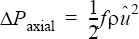whereis the pressure drop in the heat exchanger axial direction; the heat exchanger axial direction is specified by direction; f is the friction factor, given by friction_type and related parameters (see below); ρ is the density; u=rinletuaxial is the axial velocity at the minimum cross-section area; rinlet is the ratio of the inlet area to the minimum cross-section area, given by inlet_min_area_ratio; and uaxial is the axial component of the velocity.

To confine the flow to remain in the direction of the heat exchanger, pressure drops in the transverse directions of the heat exchanger component are introduced. The friction factor of these directions is scaled by transverse_friction_factor.

A volumetric heat source is added to the energy equation to model the heat source of the heat exchanger. This heat source is given by: (2)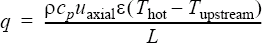where q is the heat source per volume; cP is the specific heat at constant pressure; ε is the heat exchanger effectiveness, specified by effectiveness_type and related parameters (see below); Thot is the "top water" temperature (that is, the temperature of the coolant); Tupstream is the temperature upstream of the heat exchanger, taken at a distance from the inlet surface specified by upstream_distance; and L is the heat exchanger thickness, specified by thickness. The top-water temperature is determined (at each iteration) such that the total heat flux, Q, given by:(3)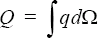is equal to the heat exchanger heat rejection, specified by coolant_heat_reject. Here Ω is the heat exchanger volume.

A constant_coolant_heat_reject heat exchanger component adds a constant heat of coolant_heat_reject to the flow passing through the heat exchanger. The coolant flow is not modeled here; only its effect on the fluid flow is modeled.

Alternatively, a constant coolant temperature can be used,
HEAT_EXCHANGER_COMPONENT( "constant coolant temp radiator" ) {
type                = constant_coolant_temperature
coolant_temperature = 350.0
...
}

The heat exchanger flow direction is given by direction, which is expressed in the global xyz coordinate system. The direction must be inward, normal to the heat exchanger inlet surface. This parameter is internally normalized.

thickness specifies the thickness (from the inlet to the outlet) of the heat exchanger. This thickness is assumed to be constant throughout the heat exchanger.

A constant friction factor applies a spatially constant friction factor. For example,
HEAT_EXCHANGER_COMPONENT( "constant friction radiator" ) {
friction_type = constant
friction      = 0.4
...
}

applies a constant friction factor of 0.4 to all the heat exchanger component elements.

The kays_london friction factor is given by:(4)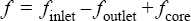where finlet, foutlet, and fcore are, respectively, the inlet effect, exit effect, and core frictions. These are:(5)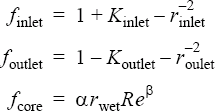Here Kinlet and Koutlet are the inlet and outlet loss coefficients, given by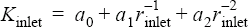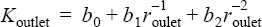The coefficients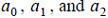are specified by inlet_loss_coefficients; the coefficients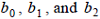are specified by outlet_loss_coefficients; rinlet is the ratio of the inlet area to the minimum cross section area, specified by inlet_min_area_ratio; and routlet is the ratio of the outlet area to the minimum cross-section area, specified by outlet_min_area_ratio.

alpha and beta are the core friction coefficients, specified by core_friction_constant and core_friction_exponent, respectively; rwet is the ratio of the wetted cross-section area to the minimum cross-section area, specified by wet_min_area_ratio; and the Reynolds number is defined by:(6)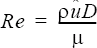where ρ is the density; u=rinletuaxial is the axial velocity at the minimum cross-section area; uaxial is the axial component of the velocity; μ is the dynamic viscosity; and D is the hydraulic diameter given by:(7)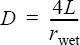L is the heat exchanger thickness, specified by thickness.

A piecewise_linear or cubic_spline type may be used to define the friction factor as a function of a single independent variable. For example,
HEAT_EXCHANGER_COMPONENT( "curve fit friction radiator" ) {
friction_type             = piecewise_linear
friction_curve_fit_values = { 0, 10.685 ;
5, 3.291 ;
10, 1.996 ;
15, 1.495 ;
20, 1.220 ;
30, 0.920 ;
40, 0.756 ;
50, 0.650 ; }
friction_curve_fit_variable = axial_velocity
...
}

defines the friction factor as a function of the axial velocity (that is, velocity in the direction of the heat exchanger). This curve fit is equivalent to the above Kays-London model, provided that the density is 1.225 and the dynamic viscosity is 1.781e-5. The friction_curve_fit_values parameter is a two-column array corresponding to the independent variable and the friction factor. The independent variable values must be in ascending order. The limit point values of the curve fit are used when friction_curve_fit_variable falls outside of the curve fit limits.

The friction_curve_fit_values data may be read from a file. For the above example, the curve fit values may be placed in a file, such as friction.fit:
0 10.685
5 3.291
10 1.996
15 1.495
20 1.220
30 0.920
40 0.756
50 0.650
HEAT_EXCHANGER_COMPONENT( "curve fit friction radiator" ) {
friction_type               = piecewise_linear
friction_curve_fit_variable = axial_velocity
...
}

A friction factor of type user_function may be used to model more complex behaviors; see the AcuSolve User-Defined Functions Manual for a detailed description of user-defined functions.

For example, consider the Kays-London friction factor of the above example. Then the input command may be given by:
HEAT_EXCHANGER_COMPONENT( "UDF friction radiator" ) {
friction_type           = user_function
friction_user_function  = "usrFrictionExample"
friction_user_values    = { 1,0,0, # flow direction
0.0629, # constant
10.6225, # proportional
-0.74 } # exponential
...
}
where the user-defined function "usrFrictionExample" may be implemented as follows:
#include "acusim.h"
#include "udf.h"
UDF_PROTOTYPE( usrFrictionExample ) ;                          /* function prototype */
Void usrFrictionExample (
UdfHd        udfHd,                                        /* Opaque handle for accessing data */
Real*        outVec,                                       /* Output vector */
Integer      nItems,                                       /* Number of elements */
Integer      vecDim                                        /* = 1 */
) {
Integer      elem ;                                        /* an element counter */
Real         aVel ;                                        /* axial velocity */
Real         coef1 ;                                       /* constant coefficient */
Real         coef2 ;                                       /* proportional coefficient */
Real         coef3 ;                                       /* exponential coefficient */
Real         xDir ;                                        /* x-component of direction vector */
Real         yDir ;                                        /* y-component of direction vector */
Real         zDir ;                                        /* z-component of direction vector */
Real*        spec ;                                        /* specie field */
Real*        usrVals ;                                     /* user values */
Real*        vel ;                                         /* velocity field */
Real*        xVel ;                                        /* x-velocity */
Real*        yVel ;                                        /* y-velocity */
Real*        zVel ;                                        /* z-velocity */
udfCheckNumUsrVals( udfHd, 6 ) ;                           /* check for error */
usrVals      = udfGetUsrVals( udfHd ) ;                    /* get the user vals */
xDir         = usrVals ;                                /* x-direction */
yDir         = usrVals ;                                /* y-direction */
zDir         = usrVals ;                                /* z-direction */
coef1        = usrVals ;                                /* const. coeff. */
coef2        = usrVals ;                                /* prop. coeff. */
coef3        = usrVals ;                                /* exp. coeff. */
vel          = udfGetElmData( udfHd, UDF_ELM_VELOCITY ) ;  /* get velocity */
xVel         = &vel[0*nItems] ;                            /* localized x-vel */
yVel         = &vel[1*nItems] ;                            /* localized y-vel */
zVel         = &vel[2*nItems] ;                            /* localized z-vel */
for ( elem   = 0 ; elem < nItems ; elem++ ) {
aVel     = xVel[elem] * xDir
+ yVel[elem] * yDir
+ zVel[elem] * zDir ;
outVec[elem] = coef1 + coef2 * pow( aVel, coef3 ) ;
}
} /* end of usrFrictionExample() */

The dimension of the returned friction vector, outVec, is the number of elements.

The friction factor in the transverse direction is controlled by transverse_friction_factor. Ideally, an infinite friction factor should be imposed in the transverse direction, resulting in a unidirectional flow through the heat exchanger. This proves, however, to be unstable. Therefore a multiple of the friction factor in the axial direction is imposed instead. The multiplier is specified by transverse_friction_factor; values from 10 to 100 are typically used.

The amount of heat transferred from the coolant to the flow depends on the effectiveness of the heat exchanger, as given in the above equations.

A constant effectiveness applies a spatially uniform effectiveness to all heat exchanger elements. For example,
HEAT_EXCHANGER_COMPONENT( "constant effectiveness radiator" ) {
effectiveness_type = constant
effectiveness      = 0.35
...
}
A piecewise_bilinear effectiveness defines a piecewise bilinear curve fit as a function of two independent variables, defined by effectiveness_curve_fit_row_variable and effectiveness_curve_fit_column_variable. The effectiveness_curve_fit_values parameter is a two dimensional array which defines the coefficients of the interpolation. The (zero , zero) entry of this array must be zero. The first row of the table defines the values of the independent column variable, while the first column of the table defines the values of the independent row variable. The values of both independent variables must be given in ascending order. For the above example, the first row (1.0000, ..., 13.500) defines the coolant_flow_rate values and the first column (0.4, ..., 3.5) defines the axial_velocity values. The values
0.7487 ... 0.0987
...
0.9452 ... 0.3329

are the effectiveness coefficients.

The coefficients may be read from a file. For the above example, the effectiveness coefficients may be placed in a file, such as effectiveness.fit:
0   1.0000 3.0000 5.0000 7.5000 10.000  13.500
0.4 0.7487 0.3302 0.2511 0.1731  0.1411  0.0987
1.1 0.8877 0.5422 0.4301 0.3021  0.2524  0.1822
1.7 0.9123 0.6321 0.5332 0.3923  0.3387  0.2487
2.6 0.9344 0.6953 0.5962 0.4693  0.4023  0.3084
3.5 0.9452 0.7233 0.6287 0.4976  0.4344  0.3329
HEAT_EXCHANGER_COMPONENT( "radiator component" ) {
...
}

An effectiveness of type user_function allows for more complex behavior; see the AcuSolve User-Defined Functions Manual for a detailed description of user-defined functions.

For example, consider the case where the effectiveness is governed by a rational function of the fluid flow. Then the input command may be given by:
HEAT_EXCHANGER_COMPONENT( "UDF effectiveness radiator" ) {
effectiveness_type           = user_function
effectiveness_user_function  = "usrEffectivenessExample"
effectiveness_user_values    = { 1,0,0, # flow direction
0.6, # coefficient 1
0.9, # coefficient 2
1.0 } # coefficient 3
...
}
where the user-defined function "usrEffectivenessExample" may be implemented as follows:
#include "acusim.h"
#include "udf.h"
UDF_PROTOTYPE( usrEffectivenessExample ) ;                       /* function prototype */
Void usrEffectivenessExample (
UdfHd          udfHd,                                        /* Opaque handle for accessing data */
Real*          outVec,                                       /* Output vector */
Integer        nItems,                                       /* Number of elements */
Integer        vecDim                                        /* = 1 */
) {
Integer        elem ;                                        /* an element counter */
Real           aVel ;                                        /* axial velocity */
Real           coef1 ;                                       /* rational function coefficient 1 */
Real           coef2 ;                                       /* rational function coefficient 2 */
Real           coef3 ;                                       /* rational function coefficient 3 */
Real           xDir ;                                        /* x-component of direction vector */
Real           yDir ;                                        /* y-component of direction vector */
Real           zDir ;                                        /* z-component of direction vector */
Real*          usrVals ;                                     /* user values */
Real*          vel ;                                         /* velocity field */
Real*          xVel ;                                        /* x-velocity */
Real*          yVel ;                                        /* y-velocity */
udfCheckNumUsrVals( udfHd, 6 ) ;                             /* check for error */
usrVals        = udfGetUsrVals( udfHd ) ;                    /* get the user vals */
xDir           = usrVals ;                                /* x-direction */
yDir           = usrVals ;                                /* y-direction */
zDir           = usrVals ;                                /* z-direction */
coef1          = usrVals ;                                /* coeff. 1 */
coef2          = usrVals ;                                /* coeff. 2 */
coef3          = usrVals ;                                /* coeff. 3 */
vel            = udfGetElmData( udfHd, UDF_ELM_VELOCITY ) ;  /* get velocity */
xVel           = &vel[0*nItems] ;                            /* localized x-vel */
yVel           = &vel[1*nItems] ;                            /* localized y-vel */
zVel           = &vel[2*nItems] ; /* localized z-vel */
for ( elem     = 0 ; elem < nItems ; elem++ ) {
aVel       = xVel[elem] * xDir
+ yVel[elem] * yDir
+ zVel[elem] * zDir ;
if ( aVel < 0 ) aVel = 0 ;
outVec[elem] = (coef1 + coef2 * aVel) / (1. + coef3 * aVel) ;
}
} /* end of usrEffectivenessExample() */

The dimension of the returned effectiveness vector, outVec, is the number of elements.

The coolant_heat_reject_multiplier_function, coolant_flow_rate_multiplier_function, friction_multiplier_function, and effectiveness_multiplier_function parameters may be used to uniformly scale the amount of heat rejected by the heat exchanger, coolant flow rate, friction factor and thermal effectiveness, respectively. The value of each of these parameters refers to the user given name of a MULTIPLIER_FUNCTION command in the input file. For example, to impose a uniform friction factor as a function of time and a bilinear effectiveness as a different function of time, the following commands may be issued:
HEAT_EXCHANGER_COMPONENT( "radiator component" ) {
shape                                   = three_node_triangle
surfaces                                = { 1, 101, 1, 9, 8 ;
2, 102, 8, 9, 5 ; }
type                                    = constant_coolant_heat_reject
coolant_heat_reject                     = 237443.5
coolant_heat_reject_multiplier_function = none
coolant_flow_rate                       = 4.6
coolant_flow_rate_multiplier_function   = none
direction                               = { 1, 0, 0 }
thickness                               = 0.1
upstream_distance                       = 0.05
friction_type                           = constant
friction                                = 0.5
friction_multiplier_function            = "time varying friction"
inlet_min_area_ratio                    = 1.296
outlet_min_area_ratio                   = 1.296
transverse_friction_factor              = 100
effectiveness_type                      = piecewise_bilinear
effectiveness_curve_fit_values          = {
0 ,  1.0000, 3.0000, 5.0000, 7.5000, 10.000, 13.500 ;
0.4, 0.7487, 0.3302, 0.2511, 0.1731,  0.1411, 0.0987 ;
1.1, 0.8877, 0.5422, 0.4301, 0.3021,  0.2524, 0.1822 ;
1.7, 0.9123, 0.6321, 0.5332, 0.3923,  0.3387, 0.2487 ;
2.6, 0.9344, 0.6953, 0.5962, 0.4693,  0.4023, 0.3084 ;
3.5, 0.9452, 0.7233, 0.6287, 0.4976,  0.4344, 0.3329 ;
effectiveness_curve_fit_row_variable    = coolant_flow_rate
effectiveness_curve_fit_column_variable = axial_velocity
effectiveness_multiplier_function       = "time varying effectiveness"
}
MULTIPLIER_FUNCTION( "time varying friction" ) {
type                                    = piecewise_linear
curve_fit_values                        = { 0, 1.0 ;
10, 1.0 ;
20, 0.5 ;
40, 0.7 ;
80, 1.2 ; }
curve_fit_variable                      = time
}
MULTIPLIER_FUNCTION( "time varying effectiveness" ) {
type                                    = piecewise_linear
curve_fit_values                        = { 0, 1.0 ;
10, 2.0 ;
20, 2.5 ;
40, 2.7 ;
80, 2.7 ; }
curve_fit_variable                      = time
}